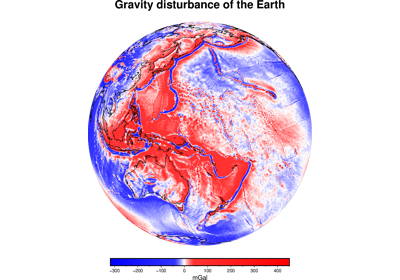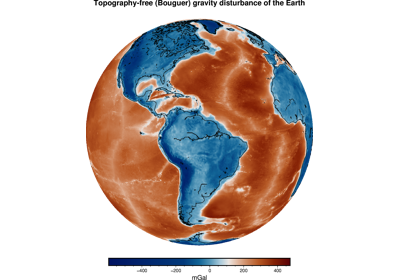# harmonica.normal_gravity¶

harmonica.normal_gravity(latitude, height)[source]

Calculate normal gravity at any latitude and height.

Computes the magnitude of the gradient of the gravity potential (gravitational + centrifugal) generated by a reference ellipsoid at the given latitude and (geometric) height. Uses of a closed form expression [LiGotze2001] and the current reference ellipsoid defined by harmonica.set_ellipsoid.

Parameters
• latitude (float or array) – The (geodetic) latitude where the normal gravity will be computed (in degrees).

• height (float or array) – The ellipsoidal (geometric) height of computation point (in meters).

Returns

gamma (float or array) – The computed normal gravity (in mGal).

## Examples using harmonica.normal_gravity¶